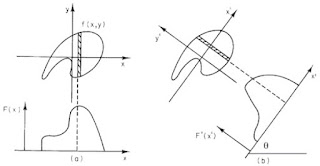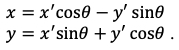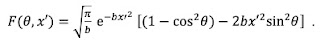Contributor profile | More stories
 Story Views Now: Last hour: Last 24 hours: Total:

# The Central Slice Theorem: An Example

The central slice theorem is key to understanding tomography. In Intermediate Physics for Medicine and Biology, Russ Hobbie and I ask the reader to prove the central slice theorem in a homework problem. Proofs are useful for their generality, but I often understand a theorem better by working an example. In this post, I present a new homework problem that guides you through every step needed to verify the central slice theorem. This example contains a lot of math, but once you get past the calculation details you will find it provides much insight.

The central slice theorem states that taking a one-dimensional Fourier transform of a projection is equivalent to taking the two-dimensional Fourier transform and evaluating it along one direction in frequency space. Our “object” will be a mathematical function (representing, say, the x-ray attenuation coefficient as a function of position). Here is a summary of the process, cast as a homework problem.

Section 12.4

Problem 21½. Verify the central slice theorem for the object

(a) Calculate the projection of the object using Eq. 12.29,

Then take a one-dimensional Fourier transform of the projection using Eq. 11.59,

(b) Calculate the two-dimensional Fourier transform of the object using Eq. 12.11a,

Then transform (kx,ky) to (θ,k) by converting from Cartesian to polar coordinates in frequency space.

(c) Compare your answers to parts (a) and (b). Are they the same?

I’ll outline the solution to this problem, and leave it to the reader to fill in the missing steps.Fig. 12.12 from IPMB, showing how to do a projection.

#### The Projection

Figure 12.12 shows that the projection is an integral of the object along various lines in the direction θ, as a function of displacement perpendicular to each line, x’. The integral becomes

Note that you must replace x and y by the rotated coordinates x’ and y’You can verify that x2 + y2= x’2 + y’2.

After some algebra, you’re left with integrals involving eby’2 (Gaussian integrals) such as those analyzed in Appendix K of IPMB. The three you’ll need are

The resulting projection isThink of the projection as a function of x’, with the angle θ being a parameter.

#### The One-Dimensional Fourier Transform

The next step is to evaluate the one-dimensional Fourier transform of the projection

The variable k is the spatial frequency. This integral isn’t as difficult as it appears. The trick is to complete the square of the exponentThen make a variable substitution u = x’ + ik2b. Finally, use those Gaussian integrals again. You getThis is our big result: the one-dimensional Fourier transform of the projection. Our next goal is to show that it’s equal to the two-dimensional Fourier transform of the object evaluated in the direction θ.

#### Two-Dimensional Fourier Transform

To calculate the two-dimensional Fourier transform, we must evaluate the double integral

The variables kx and ky are again spatial frequencies, and they make up a two-dimensional domain we call frequency space.

You can separate this double integral into the product of an integral over x and an integral over y. Solving these requires—guess what—a lot of algebra, completing the square, and Gaussian integrals. But the process is straightforward, and you get#### Select One Direction in Frequency Space

If we want to focus on one direction in frequency space, we must convert to polar coordinates: kx = k cosθ and ky = k sinθ. The result is

This is exactly the result we found before! In other words, we can take the one-dimensional Fourier transform of the projection, or the two-dimensional Fourier transform of the object evaluated in the direction θ in frequency space, and we get the same result. The central slice theorem works.

I admit, the steps I left out involve a lot of calculations, and not everyone enjoys math (why not?!). But in the end you verify the central slice theorem for a specific example. I hope this helps clarify the process, and provides insight into what the central slice theorem is telling us.

Source: http://hobbieroth.blogspot.com/2021/08/the-central-slice-theorem-example.html

Before It’s News® is a community of individuals who report on what’s going on around them, from all around the world.

Anyone can join.
Anyone can contribute.
Anyone can become informed about their world.

Order by Phone at 888-809-8385 or online at https://mitocopper.com M - F 9am to 5pm EST

Order by Phone at 888-388-7003 or online at https://www.herbanomic.com M - F 9am to 5pm EST

Order by Phone at 888-388-7003 or online at https://www.herbanomics.com M - F 9am to 5pm EST

Humic & Fulvic Trace Minerals Complex - Nature's most important supplement! Vivid Dreams again!

- Improve immune system health and reduce inflammation

Ultimate Clinical Potency Curcumin - Natural pain relief, reduce inflammation and so much more.

MitoCopper - Bioavailable Copper destroys pathogens and gives you more energy. (See Blood Video)
Oxy Powder - Natural Colon Cleanser!  Cleans out toxic buildup with oxygen!
Nascent Iodine - Promotes detoxification, mental focus and thyroid health.
Smart Meter Cover -  Reduces Smart Meter radiation by 96%!  (See Video)

Immusist Beverage Concentrate - Proprietary blend, formulated to reduce inflammation while hydrating and oxygenating the cells.